Question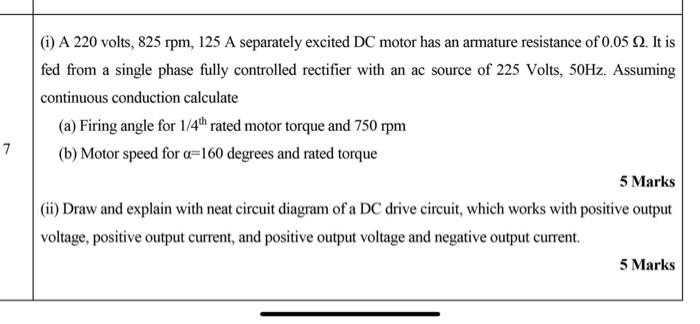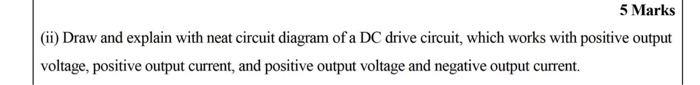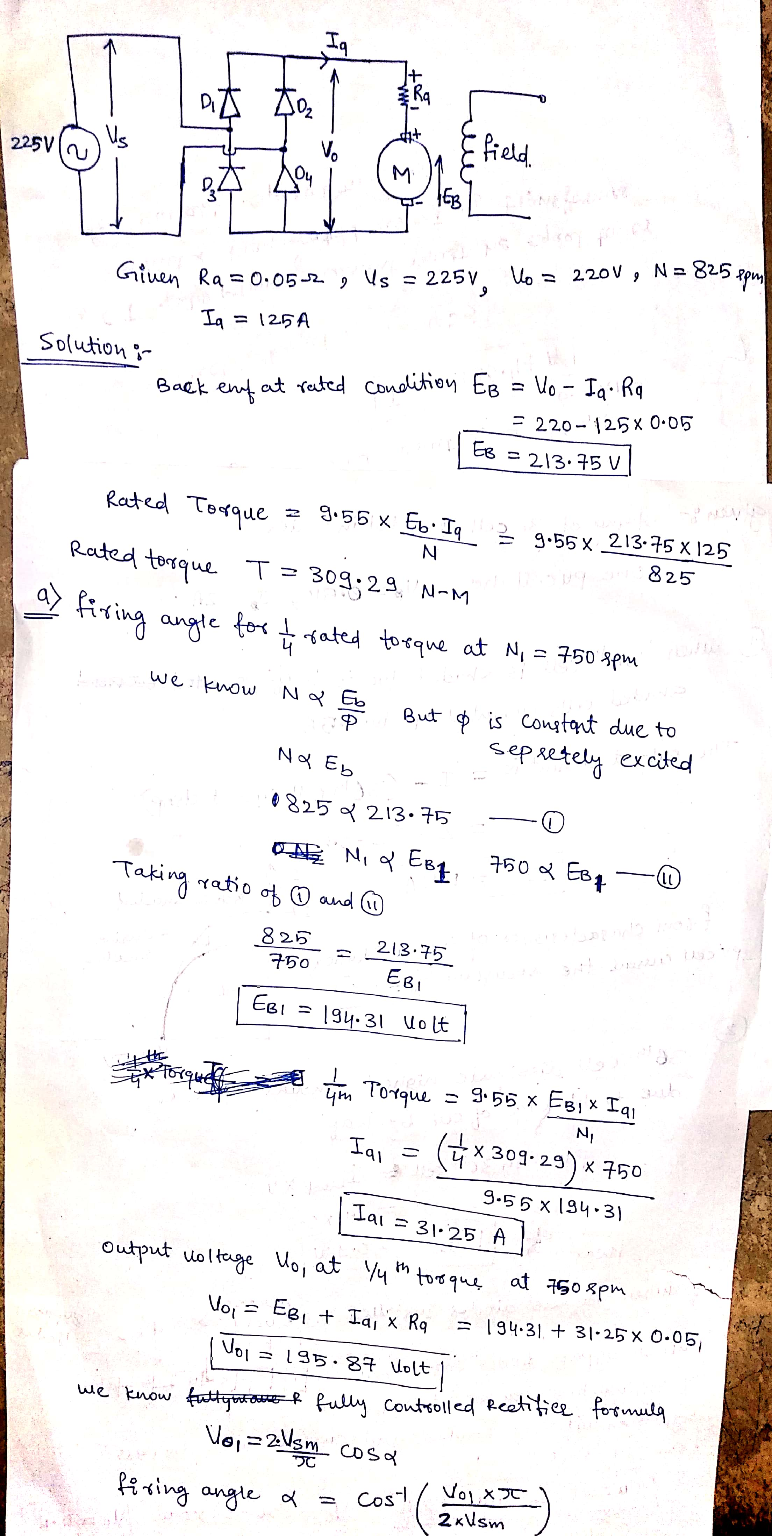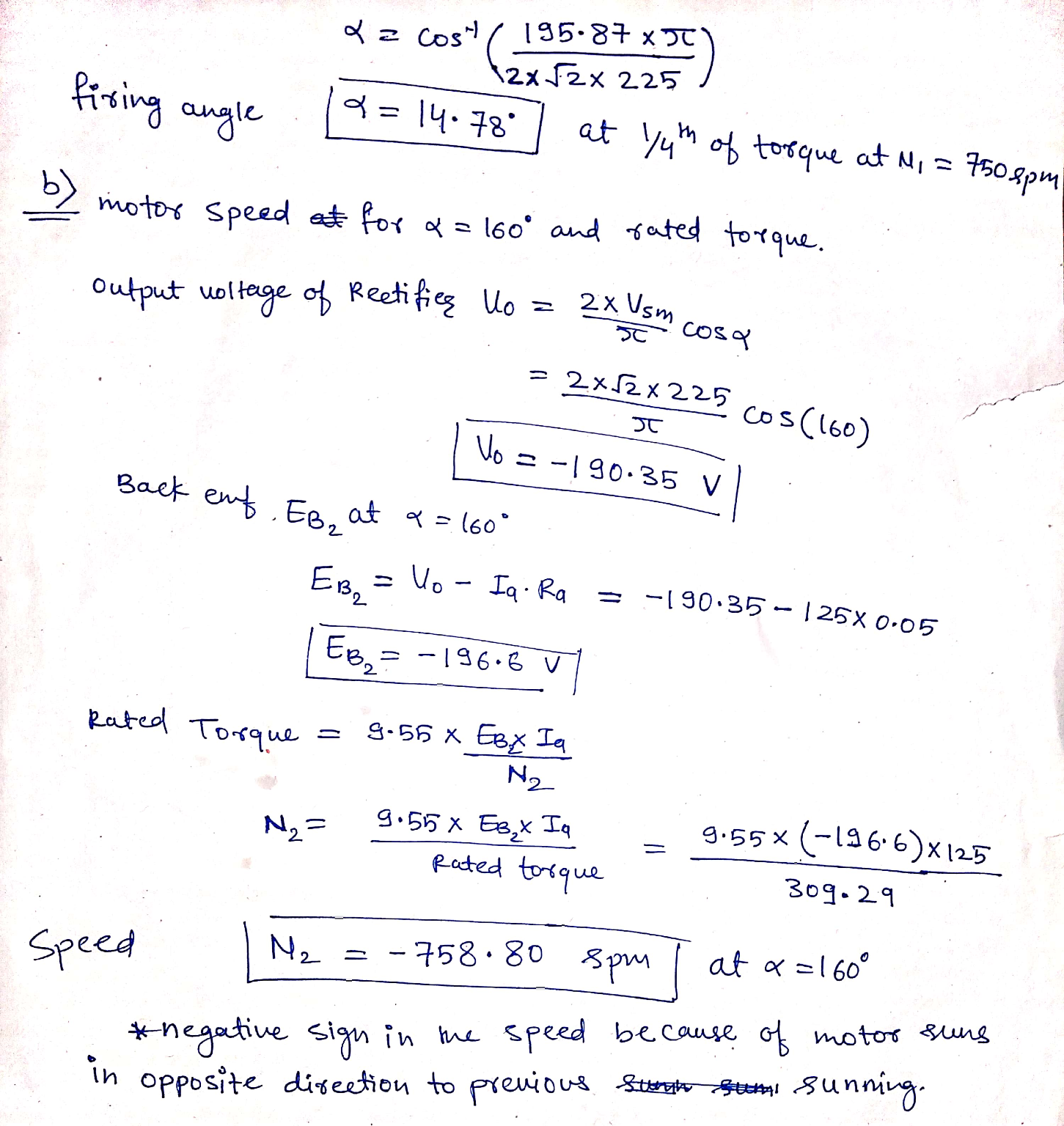#### Earn Coins

Coins can be redeemed for fabulous gifts.

Similar Homework Help Questions
• ### A 230 V, 1000 rpm, 30 A separately excited motor has armature resistance and inductance of...

A 230 V, 1000 rpm, 30 A separately excited motor has armature resistance and inductance of 0.7 Ω and 50 mH respectively. Motor is controlled in regenerative braking by a chopper operating at 800 Hz from a dc source of 230 V. Assuming continuous conduction a) Calculate duty ratio of chopper for rated torque and the speed of 800 rpm. b) What will be the motor speed for duty ratio of 0.6 and rated motor torque? c) What will be...

• ### solve it now please A 220 V, 1000 rpm, separately excited dc motor is fed from...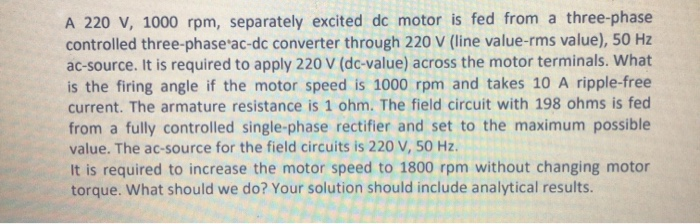solve it now please A 220 V, 1000 rpm, separately excited dc motor is fed from a three-phase controlled three-phase ac-dc converter through 220 V (line value-rms value), 50 Hz ac-source. It is required to apply 220 V (dc-value) across the motor terminals. What is the firing angle if the motor speed is 1000 rpm and takes 10 A ripple-free current. The armature resistance is 1 ohm. The field circuit with 198 ohms is fed from a fully controlled single-phase...

• ### 3) A saperately excited DC motor has the following parameters: Ra = 10 Ke = 0.4...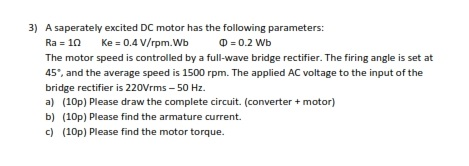3) A saperately excited DC motor has the following parameters: Ra = 10 Ke = 0.4 V/rpm. Wb O = 0.2 Wb The motor speed is controlled by a full-wave bridge rectifier. The firing angle is set at 45, and the average speed is 1500 rpm. The applied AC voltage to the input of the bridge rectifier is 220Vrms - 50 Hz. a) (10p) Please draw the complete circuit. (converter + motor) b) (10p) Please find the armature current. c)...

• ### 3) A saperately excited DC motor has the following parameters: Ra = 10 Ke = 0.4...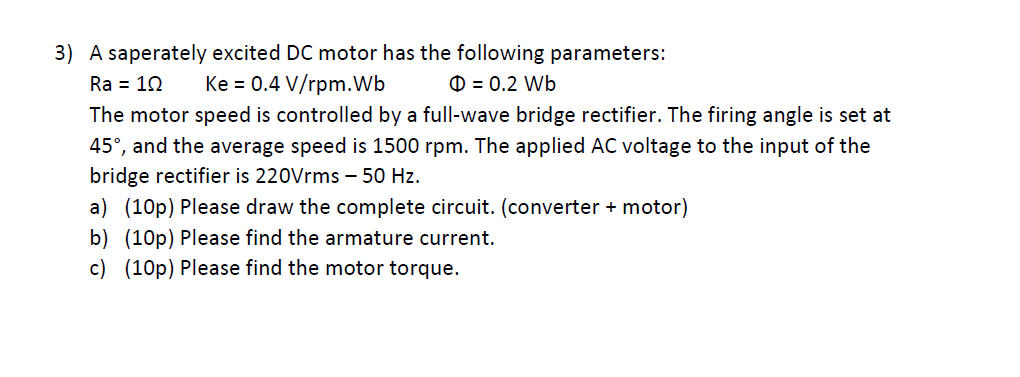3) A saperately excited DC motor has the following parameters: Ra = 10 Ke = 0.4 V/rpm. Wb 0 = 0.2 Wb The motor speed is controlled by a full-wave bridge rectifier. The firing angle is set at 45°, and the average speed is 1500 rpm. The applied AC voltage to the input of the bridge rectifier is 220Vrms - 50 Hz. a) (10p) Please draw the complete circuit. (converter + motor) b) (10p) Please find the armature current. c)...

• ### A power electronic converter used to drive a separately-excited DC motor for application in Sohar, Oman...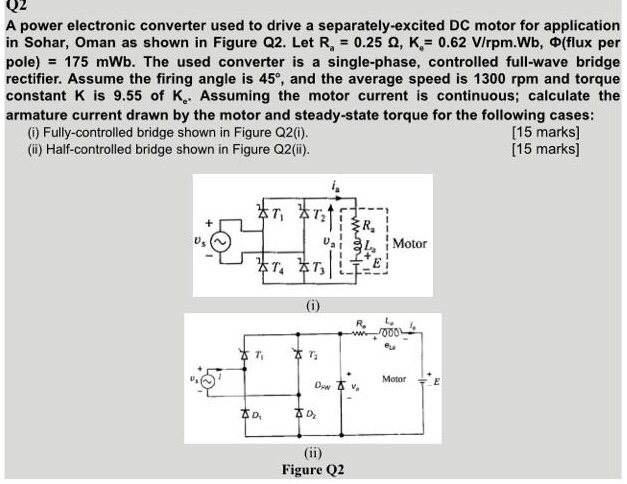A power electronic converter used to drive a separately-excited DC motor for application in Sohar, Oman as shown in Figure Q2. Let R = 0.25 , K = 0.62 V/rpm.Wb, (flux per pole) = 175 mWb. The used converter is a single-phase, controlled full-wave bridge rectifier. Assume the firing angle is 45°, and the average speed is 1300 rpm and torque constant K is 9.55 of K. Assuming the motor current is continuous; calculate the armature current drawn by the...

• ### i. The speed of a separately excited dc motor is controlled by a one-phase full-wave converter. The field circuit is also controlled by a full-wave converter, see V supply) Vs pk-150V Ra-0.310 L Rf 1...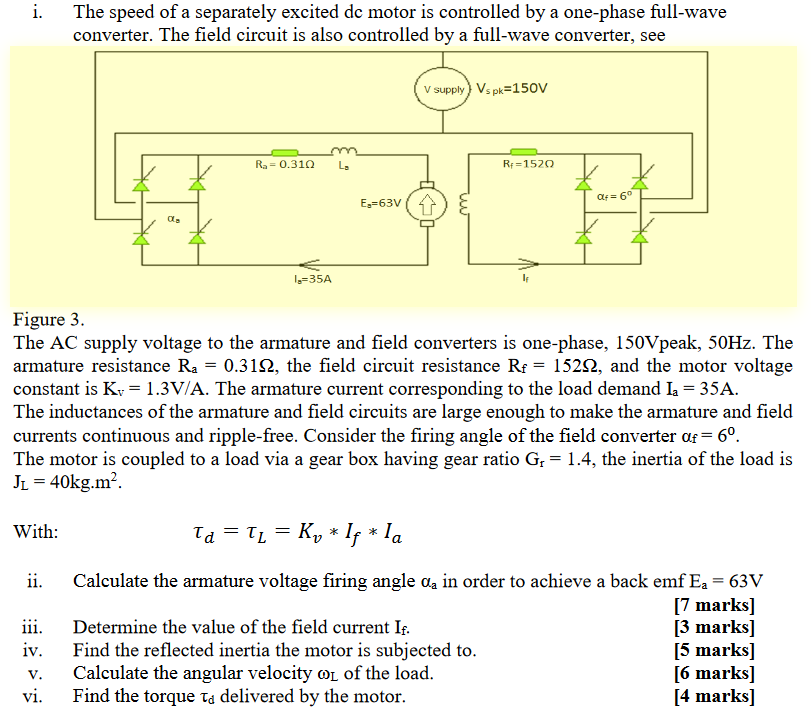i. The speed of a separately excited dc motor is controlled by a one-phase full-wave converter. The field circuit is also controlled by a full-wave converter, see V supply) Vs pk-150V Ra-0.310 L Rf 152O E-63V α. 1-35A Figure 3 The AC supply voltage to the armature and field converters is one-phase, 150Vpeak, 50Hz. The armature resistance Ra-0.31Ω, the field circuit resistance Rf-152Ω, and the motor voltage constant is Kv-1.3V/A. The armature current corresponding to the load demand la-35A The...

• ### 3. A controlled single-phase fiull-wave controlled rectifier is connected in a de motor drive in Figure...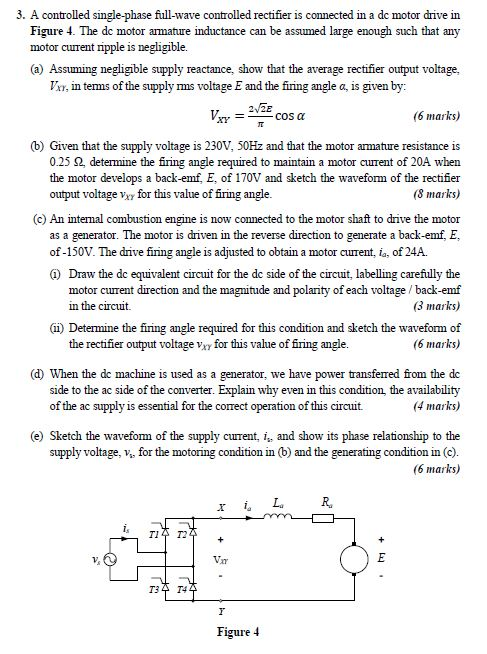3. A controlled single-phase fiull-wave controlled rectifier is connected in a de motor drive in Figure 4. The de motor armature inductance can be assumed large enough such that any motor curent ripple is negligible. (a) Assuming negligible supply reactance, show that the average rectifier output voltage V, in tems of the supply ms voltage E and the firing angle a, is given by 2/2E (6 marks) cos a _ (b) Given that the supply voltage is 230V, 50HZ and...

• ### Open Book Exam A 220 V, 1000 V dc-sourc rpm, separately dc motor is fed from a dc-dc converter th...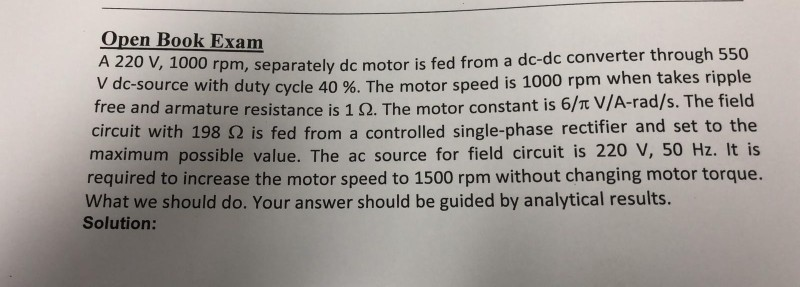Open Book Exam A 220 V, 1000 V dc-sourc rpm, separately dc motor is fed from a dc-dc converter through 550 e with duty cycle 40 %. The motor speed is 1000 rpm when takes ripple free and armature resistance is 1 Ω. The motor constant is 6/π V/A-rad/s. The field circuit with 198 Ω is fed from a controlled single-phase rectifier and set to the maximum possible value. The ac source for field circuit is 220 V, 50 Hz....

• ### 6. The speed of a 150 HP, 650 V, 1750 rpm, separately excited DC motor is...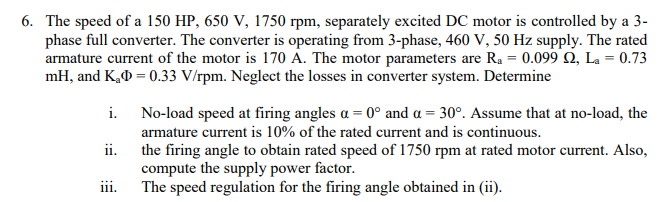6. The speed of a 150 HP, 650 V, 1750 rpm, separately excited DC motor is controlled by a 3- phase full converter. The converter is operating from 3-phase, 460 V, 50 Hz supply. The rated armature current of the motor is 170 A. The motor parameters are Ra = 0.099 2, La = 0.73 mH, and K.D=0.33 V/rpm. Neglect the losses in converter system. Determine i. No-load speed at firing angles a = 0º and a = 30°. Assume...

• ### 3. A 20kW, 250V, 1000rpm shunt excited dc motor has armature and field resistances of 0.2...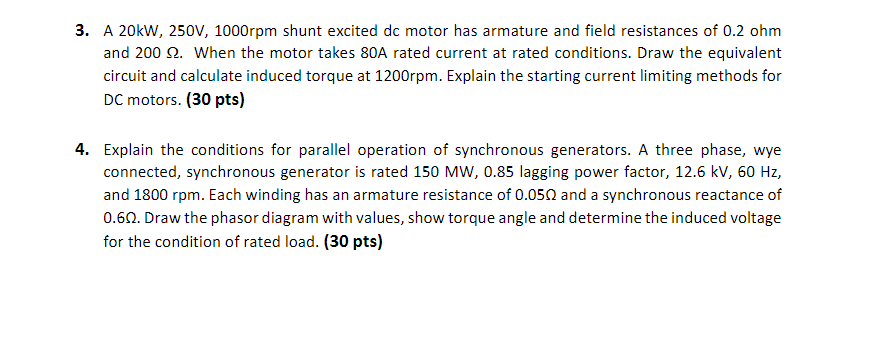3. A 20kW, 250V, 1000rpm shunt excited dc motor has armature and field resistances of 0.2 ohm and 2002. When the motor takes 80A rated current at rated conditions. Draw the equivalent circuit and calculate induced torque at 1200rpm. Explain the starting current limiting methods for DC motors. (30 pts) 4. Explain the conditions for parallel operation of synchronous generators. A three phase, wye connected, synchronous generator is rated 150 MW, 0.85 lagging power factor, 12.6 kV, 60 Hz, and...